# Ellipse Detection/Localization

In this article I’ll discuss multiple ways to localize an ellipse in an image.

#### “DUAL CONIC” method

This method is from Hebert09. I think it’s akin to the “opencv” checker localization algorithm in that it’s a linear algorithm that operates on the image gradients.

Anyway, to understand this method, you need to understand what a conic section is. A conic section is a curve obtained as the intersection of the surface of a cone with a plane. The possible conic sections are a hyperbola, parabola, and ellipse. It turns out that a conic can be represented as a matrix: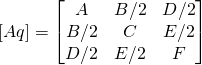and points, represented in homogeneous coordinates as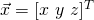, lie on the conic if: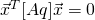Now, it turns out there’s a “dual” to this in that a line, represented in homogeneous coordinates as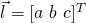, is tangent to the conic if: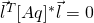Where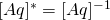.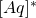is known as the “dual conic”.

Proving this is a little mathy, so I’ll just leave this as a “black box” for now, but there are plenty of resources out there that explain why this is the case. Anyway, Hebert09 made the observation that lines orthogonal to the image gradients should be tangent to the ellipse, as shown below:

So these lines will satisfy the dual conic constraint. In addition to this, the dual conic constraint is linear, so it can be solved with a linear solver.

The first thing to note is the homogeneous form for the point slope formula is: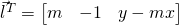Where m is the slope and x and y are a point on the line. The slope of the gradient is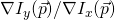. The orthogonal slope is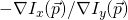. We then obtain: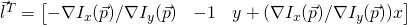If you multiple through by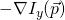, we arrive at:These lines must satisfy. If you multiply this out, you obtain: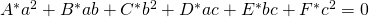We can form the matrix equation: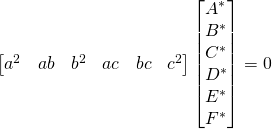From, it’s apparent thatcan have any arbitrary scaling, so an additional constraint needs to be added. According to Hebert09, a constraint of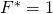ensures the conic is an ellipse. If applied, this results in:Now, we can use a linear solver to solve for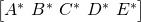and hence. The original conic,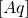, which is what we’re really after, can be recovered by taking the inverse of.

I guess the last thing to do is to convert the conic to a more tractable form which usually includes the center point, minor and major axes, and the rotation of the major axis. For that, I used this stack overflow post.

If you’d like to see an example in action, you can download my camera calibration toolbox. The functions which implements this are alg.fit_conic(), alg.refine_ellipse_dualconic(), and alg.conic2ellipse() and examples can be found in the unit tests folder.

#### “Edges” method

I tried to make an analogous function to the “edges” method from the checker detection article. If we revisit the equation used for the checker method, it turns out that the first term of the equation is equivalently: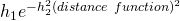Where:

•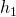– magnitude of the gradient peak
•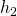– variance (i.e. width) of the gaussian distribution

This “distance function” is easy to compute for lines. But, for ellipses, it’s quite difficult. After googling around for a bit, I couldn’t find any satisfactory solutions (or any non-iterative closed form solutions which are differentiable; if a solution does exist, please leave a comment). So, I decided to use an approximation to the distance function as shown below:

Computing distance this way is reasonable as long as the minor axis isn’t super small compared to the major axis (i.e. the ellipse is still “circle-ish”). You can compute this by starting out with the general equation for an ellipse: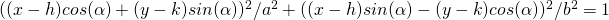I plugged this into matlab’s symbolic toolbox and then substituted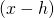for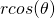and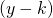for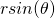. Then, I substitutedfor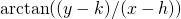. I then solve()‘d for the roots,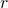. If you select the positive root,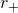, it represents a function where anycoordinate will return the distance from the center of the ellipse to the edge of the ellipse on a line which goes through. To compute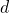, all you need is the distance from the center of the ellipse to the point, and then subtractfrom this value. This will be theshown in the figure above, which, again, is an approximation to the distance function.

After computing the derivatives symbolically (and getting a pretty massive set of ugly equations), optimization is possible. Below is an example of the optimization process:

Note that the final optimized edge function actually matches the array gradient for an ellipse very well.

If you’d like to see an example in action, you can download my camera calibration toolbox. The function which implements it is alg.refine_ellipse_edges() and an example can be found in the unit tests folder.

#### “dot” method

I wanted to try to model an ellipse directly instead of using the image gradients like in the “dualconic” and “edges” methods. It turns out you can use a sigmoid-like function to do this. The specific function I needed was a step function convolved with a gaussian. It turns out this function exists and is called the “error function” (or erf). The function can be constructed similar to the “edges” method: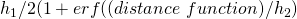Where:

•– magnitude of edge peak
•– “blur” of edge

I used the same distance function approximation in the “edges” method section and just plugged it into the equation above. Again, to make things easy, I computed the derivatives symbolically and then was able to use nonlinear optimization. Below is an example of the optimization process:

The optimized “dot” function matches the array magnitude pretty well. The downsides with this method are: you have to know beforehand if the dot is black and the background is white (or vice-versa) and it will be affected by non-uniform lighting. These issues aren’t a problem with the gradient based approaches. However, not having to compute the gradient also has it’s advantages, as sometimes computing gradients can be unstable if the image is resampled to a higher resolution.

If you’d like to see an example in action, you can download my camera calibration toolbox. The function which implements it is alg.refine_ellipse_dot() and an example can be found in the unit tests folder.

#### Gradient ascent with backtracking method

This method maximizes the sum of sampled points along an ellipse on the array gradient magnitude. For example:

The cost we want to maximize is:Where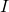is the image gradient magnitude and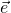is a parameterization of an ellipse. To maximize it, I’ll use gradient ascent with backtracking. Backtracking is a robust way to ensure the cost function increases at every iteration. In addition, it provides a bounded way to ensure that larger steps result in a larger increase in cost. This acts to ensure the nearest-ish local maximum is found, which may be good or bad depending on the application. For this ellipse fitting technique, it’s assumed a reasonable initial guess is provided, so we actually do want to fit to the nearest-ish local maximum.

The figure below gives a good intuition for backtracking:

The backtracking condition is to pick a smaller step if the following condition exists: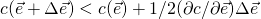From the figure above, that means if the cost computed at the next step is below the second dashed line, then the step size is decreased. The decrease is done by multiplying the previous step size by a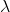parameter which is less than 1.

For our case, the step is selected to be equal to the gradient times aparameter: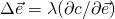So the condition becomes: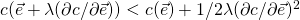To compute the gradient,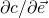, we first need to parameterize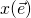and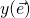: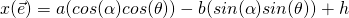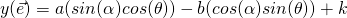where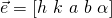. For, I chose to use 100 sampling points. The gradient is then: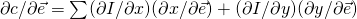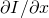andare computed from the gradient of the image gradient magnitude and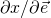and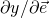are computed by taking the partial derivatives of the parameterizations ofandin the equations above.

An example of the optimization is shown below:

This is kind of an extreme case, since the initial guess is poor and the ellipse has a large aspect ratio, but after quite a few iterations (70) it does appear to fit the array gradient magnitude pretty well, which demonstrates the robustness of the method.

If you’d like to see an example in action, you can download my camera calibration toolbox. The function which implements this is alg.fp_detect_blobs() and an example can be found in the unit tests folder.